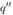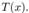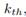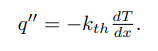### Create an Account

Home / Questions / Consider an experiment where a researcher is attempting to measure the thermal conductivit...

# Consider an experiment where a researcher is attempting to measure the thermal conductivity of a copper bar The researcher applies a heat input 00 to a copper bar and uses four thermos couples to me

Consider an experiment where a researcher is attempting to measure the thermal conductivity of a copper bar. The researcher applies a heat input00 to a copper bar and uses four thermos couples to measure the local bar temperatureThe thermal conductivity,, can be calculated from the equationVariables associated with the experiment are the (a) thermal conductivity of the bar, (b) heater input, (c) temperature of points 1, 2, 3, and 4 from the thermocouples, (d) pressure and temperature of the surrounding air, (e) smoothness of copper bar at the interfaces with the heaters, and (f) position of the thermocouples. Determine whether each variable is dependent, independent, or extraneous. Then determine whether each variable is a parameter or a measurand.

Jul 26 2020 View more View LessSubscribe To Get Solution